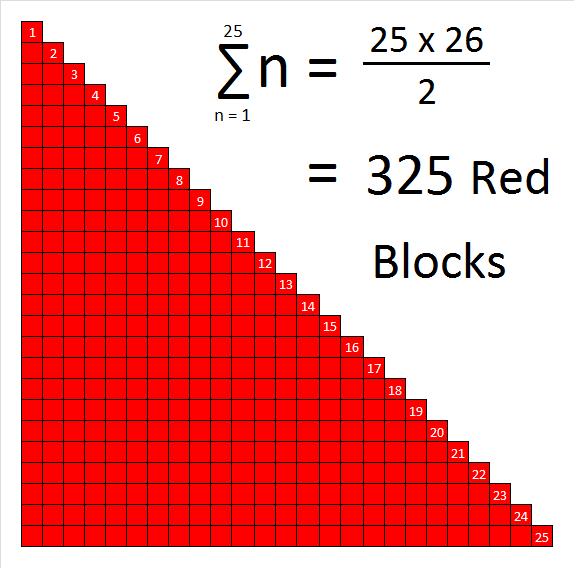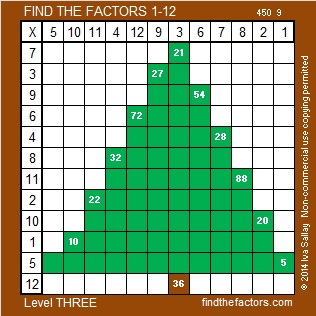# 455 and Level 6

I don’t mean to sound greedy, but if there were 13 days of Christmas instead of only 12, my true love would give me 455 gifts instead of only 364. That’s because the sum of the first 13 triangular numbers is 455. Come on, that’s 91 more gifts. Funny thing, 91 is one of the factors of 455. Also, I know I’m not the first person to notice that (13 x 14 x 15)/6 = 455. As I’m sure you can see, 455 is a fabulous tetrahedral number.Print the puzzles or type the factors on this excel file:  12 Factors 2015-04-06

——————————————————————————————————————-

• 455 is a composite number.
• Prime factorization: 455 = 5 x 7 x 13
• The exponents in the prime factorization are 1, 1, and 1. Adding one to each and multiplying we get (1 + 1)(1 + 1)(1 + 1) = 2 x 2 x 2 = 8. Therefore 445 has exactly 8 factors.
• Factors of 455: 1, 5, 7, 13, 35, 65, 91, 455
• Factor pairs: 455 = 1 x 455, 5 x 91, 7 x 65, or 13 x 35
• 455 has no square factors that allow its square root to be simplified. √455 ≈ 21.3307——————————————————————————————————————-# 325 is a Triangular Number

• 325 is a composite number.
• Prime factorization: 325 = 5 x 5 x 13, which can be written 325 = 5² x 13
• The exponents in the prime factorization are 2 and 1. Adding one to each and multiplying we get (2 + 1)(1 + 1) = 3 x 2  = 6. Therefore 325 has exactly 6 factors.
• Factors of 325: 1, 5, 13, 25, 65, 325
• Factor pairs: 325 = 1 x 325, 5 x 65, or 13 x 25
• Taking the factor pair with the largest square number factor, we get √325 = (√13)(√25) = 5√13 ≈ 18.028325 is a triangular number. 1 + 2 + 3 + 4 + . . . + 22 + 23 + 24 + 25 = 325. Shorthand for that sum of 25 numbers is given with the ∑ sign in the graphic below. The way to find the sum quickly using multiplication is also given:If you look at this Level 3 puzzle from far enough away, you also might see a triangle!Print the puzzles or type the factors on this excel file: 12 Factors 2014-12-15

A Logical Approach to FIND THE FACTORS: Find the column or row with two clues and find their common factor. Write the corresponding factors in the factor column (1st column) and factor row (top row).  Because this is a level three puzzle, you have now written a factor at the top of the factor column. Continue to work from the top of the factor column to the bottom, finding factors and filling in the factor column and the factor row one cell at a time as you go.